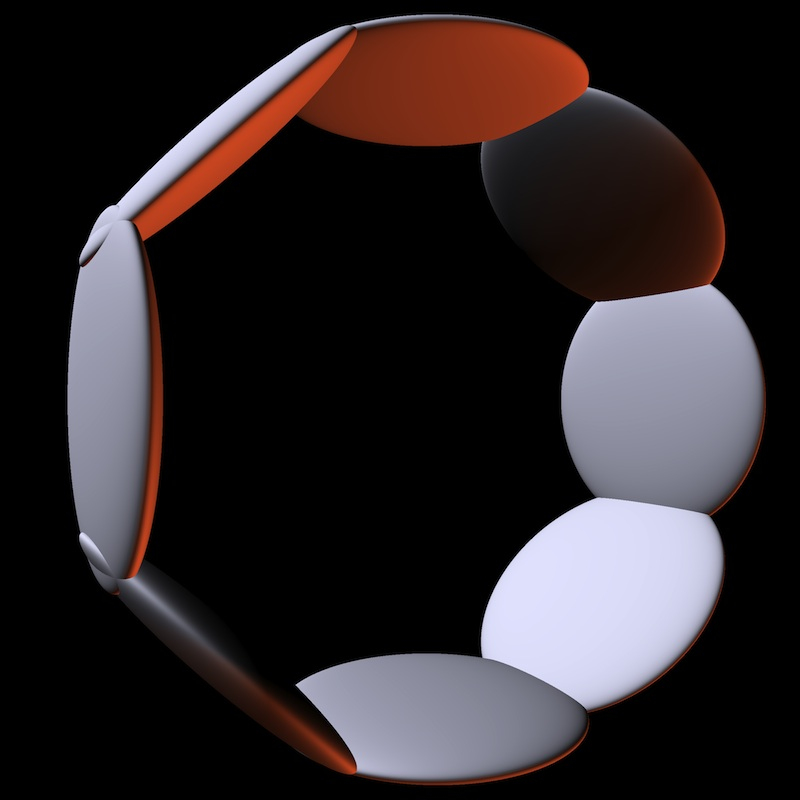## A simple technique for computing the axis-aligned bounding box of an ellipsoid

Here is a simple technique for computing the tight axis-aligned bounding box (AABB) of an ellipsoid at any orientation. The technique assumes that the ellipsoid is modeled by transforming a zero-centered unit-radius sphere using an affine transformation, of which we know the corresponding matrix $$M$$. This is often the case in a ray-tracing application. Here, the translation is not important so for simplicity we assume that $$M$$ is a $$3\times3$$ matrix. tl/dr: see the last paragraph.
--
Samuel Hornus, February 7, 2013.

#### Transforming normals

As is well known (and proving it is an easy exercice), when transforming an object affinely using matrix $$M$$, the normals should be transformed using the cofactor matrix of $$M$$ which is proportional to the transpose of the inverse of $$M$$. (Don't forget to re-normalize your normal, if needed, after it's been transformed.)

#### The AABB of an ellipsoid

Let $$E$$ be our ellipsoid. Since $$E$$ is convex and smooth, there is a bijection between the direction of the normal and the points on the surface of $$E$$. The bijection is not trivial for the ellipsoid, but it is trivial for the unit sphere from which $$E$$ is modeled. So we'll go through that space. In order to find the point $$q$$ of $$E$$ which is extremal along some direction $$v$$, we first transform $$v$$, which is the normal to $$E$$ at $$q$$, to the unit sphere space, i.e., we compute $$n=M^{T}v$$ where the superscript $${}^{T}$$ denotes the transpose. This gives us the normal in the local coordinate system. We then normalize $$n$$ to find the point $$p=\frac{n}{||n||}$$ of the unit sphere whose normal has direction $$n$$. ($$||\cdot||$$ is the $$\ell_2$$ norm.) Then we transform $$p$$ back to the ellipsoid space: $$q=Mp$$ is the point on the ellipsoid with normal $$v$$. In particular, the top-most point of ellipsoid $$E$$ (that with largest z-coordinate) is $$q=M\frac{M^T v}{||M^T v||}$$ with $$v=(0,0,1)^T$$.

Repeat for the x- and y- direction, then by symmetry and using the center of $$E$$, you have found the 6 intersection points of $$E$$ with its tight axis-aligned bounding box. Note that all three operations (along x-, y- and z-axes) can be done together using a matrix-matrix multiplication: simply compute the product $$MM'$$ where $$M'$$ is $$M^T$$ with its columns independently normalized.

#### Optimization

The above computes the extremal points of $$E$$ in the canonical axes directions. But the bounding box just needs the z-coordinate of the extremal point along the z-axis, and similarly for the x- and y-axes. That is, we only need the diagonal elements of $$MM'$$, which are simply the $$\ell_2$$ norms of each row of $$M$$:

Let $$c$$ be the center of $$E$$ and $$r_i$$ the $$i$$-th row of $$M$$. Then the lexicographically smallest point of the bounding box of $$E$$ is $$\text{lo}=c-\Delta.$$ Its lexicographically largest point is $$\text{hi}=c+\Delta$$ where $$\Delta=(||r_0||,||r_1||,||r_2||)^T.$$